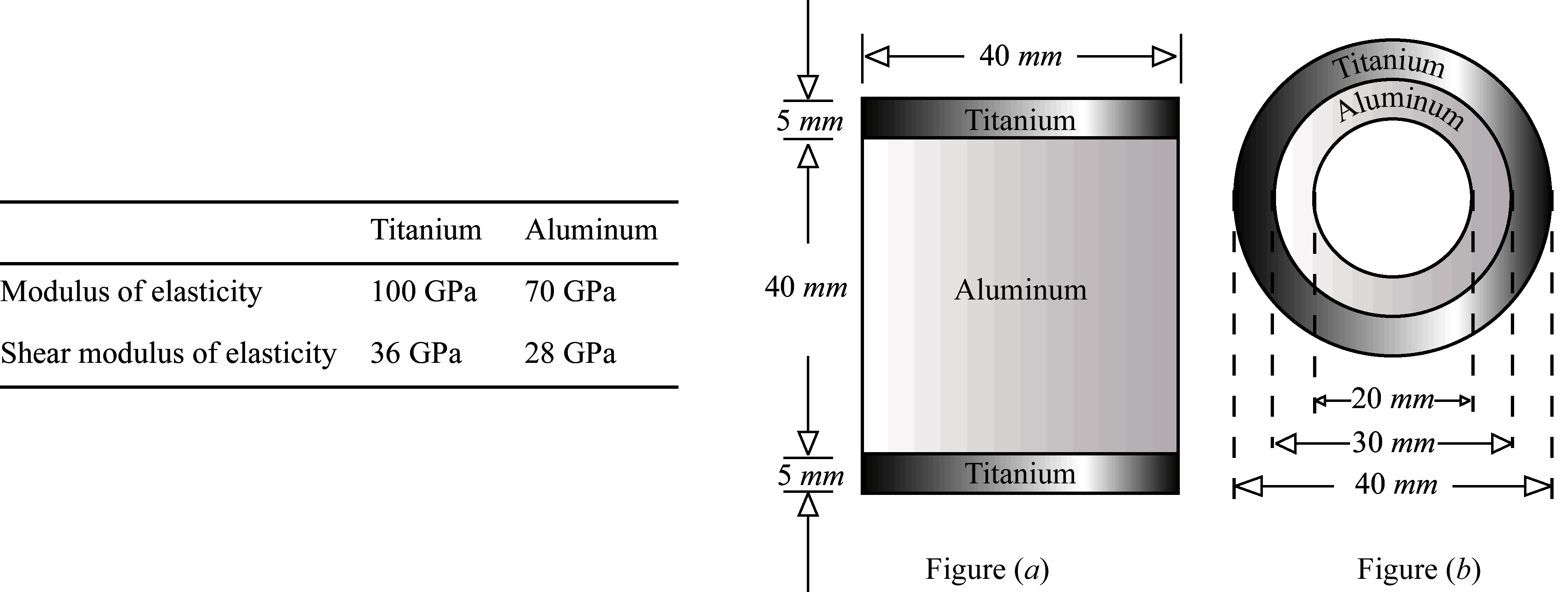# 4.2 – STRESS AND STRAIN IN COMPOSITE STRUCTURAL MEMBERS

Using the information below, choose the correct answer to the closest 3 significant figures.Name Email
1.

In Figure (a) the maximum axial strain in titanium was found to be 0.0004. The maximum axial strain in aluminum is:

2.

In Figure (a) the maximum bending normal strain in titanium was found to be 0.0004. The maximum bending normal strain in aluminum is

3.

In Figure (b) the maximum torsional shear strain in titanium was found to be 0.0004. The maximum torsional shear strain in aluminum is:

4.

In Figure (a) the maximum axial strain in aluminum was found to be 0.0003. The maximum axial stress in titanium is:

5.

In Figure (a) the maximum bending normal strain in aluminum was found to be 0.0003. The maximum bending normal stress in titanium is:

6.

In Figure (b) the maximum torsional shear strain in aluminum was found to be 0.0003. The maximum torsional shear stress in titanium is

7.

The internal axial force acting on the cross section in Figure (a) is 76 kN (T). The maximum axial stress in aluminum is:

8.

The internal torque acting on the cross section Figure (b) is 3.8 kN-m. The maximum torsional shear stress in aluminum is:

9.

The internal bending moment acting on the cross section in Figure (a) is 2.5 kN-m. The maximum bending normal stress in aluminum is

10.

The internal bending shear force acting on the cross section in Figure (a) is 50 kN. The maximum bending shear stress in aluminum is: